Chapter 3 Class 10 Pair of Linear Equations in Two Variables

Class 10
Important Questions for Exam - Class 10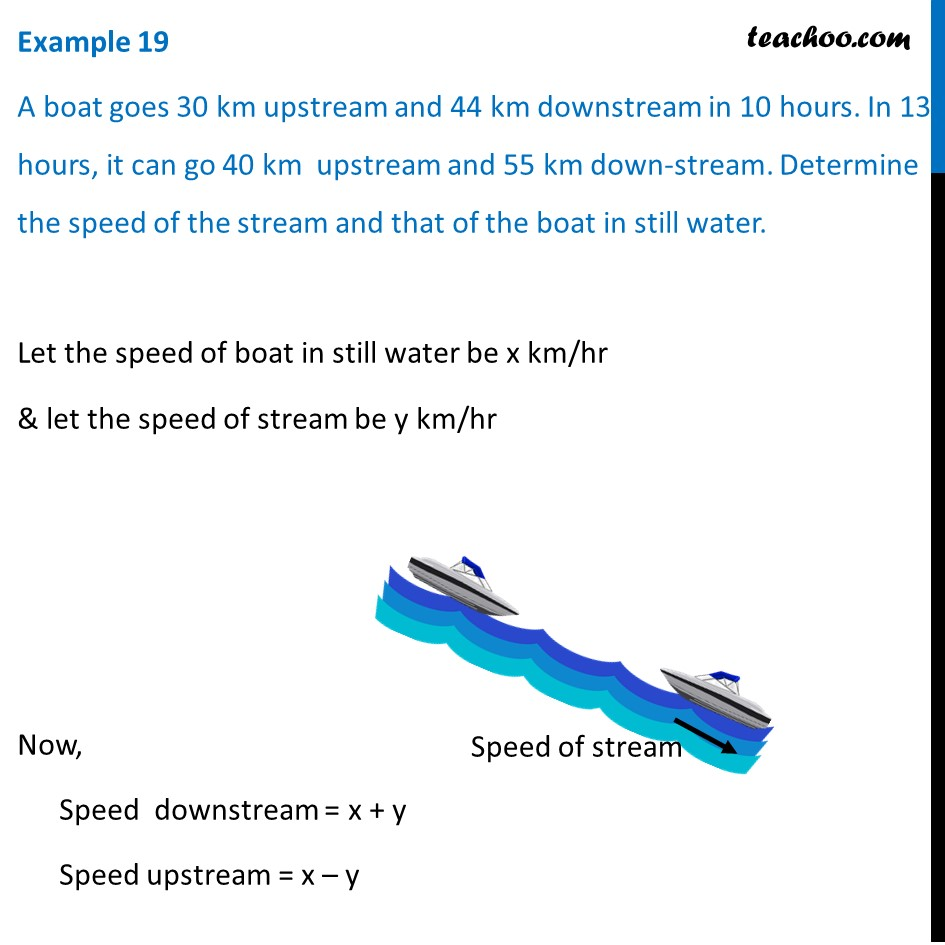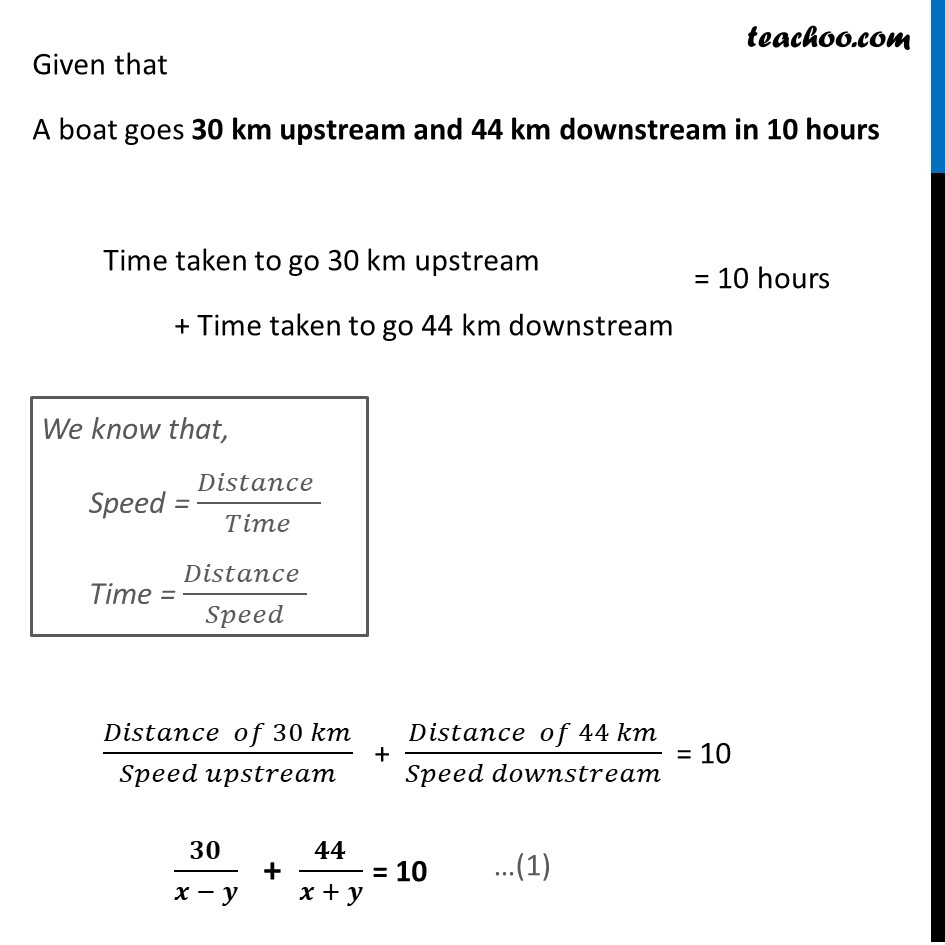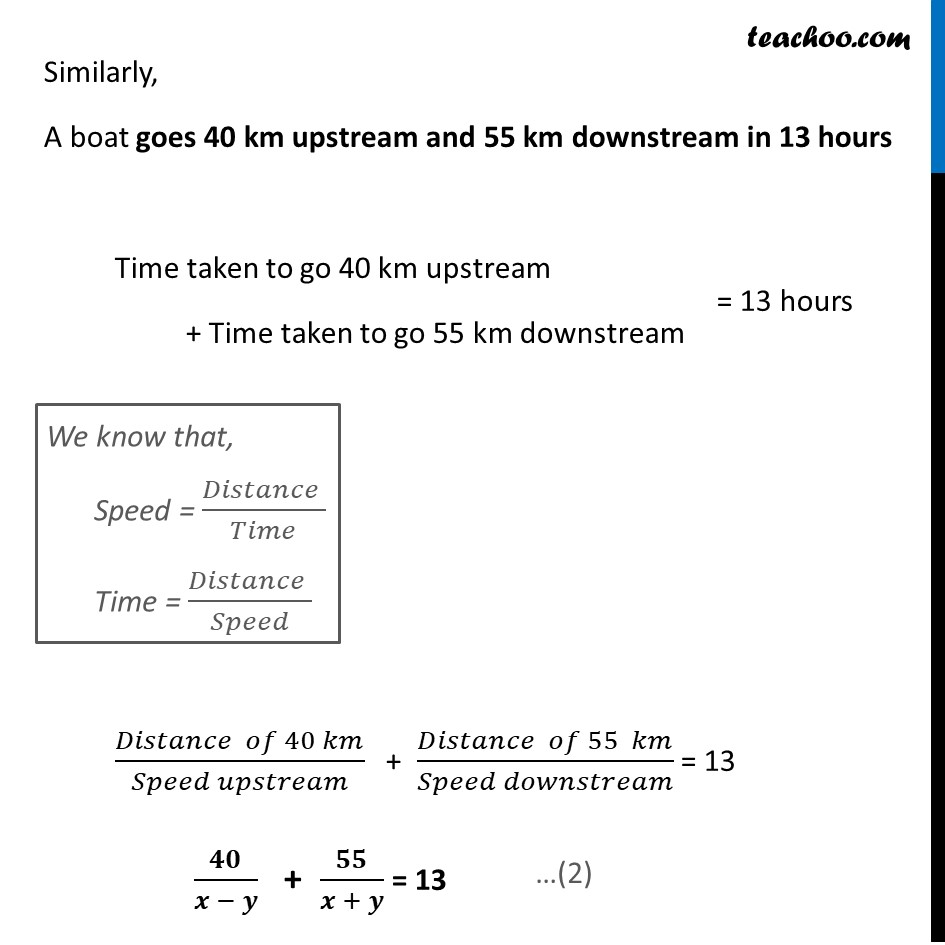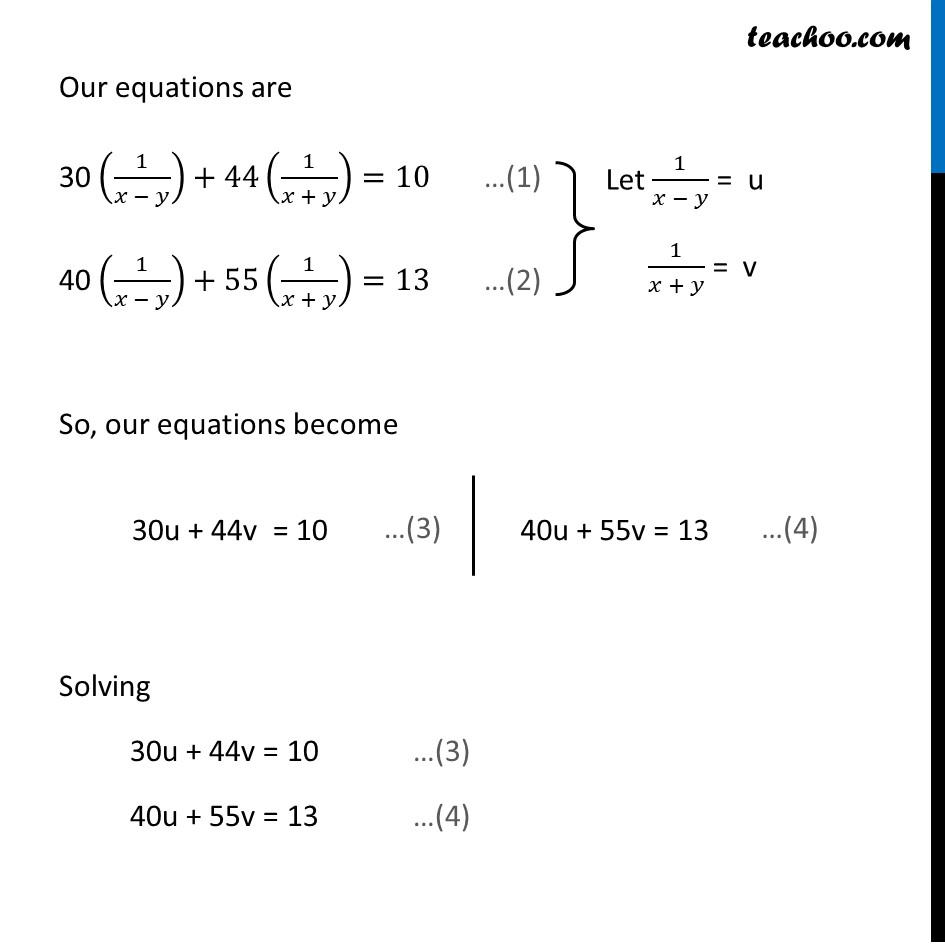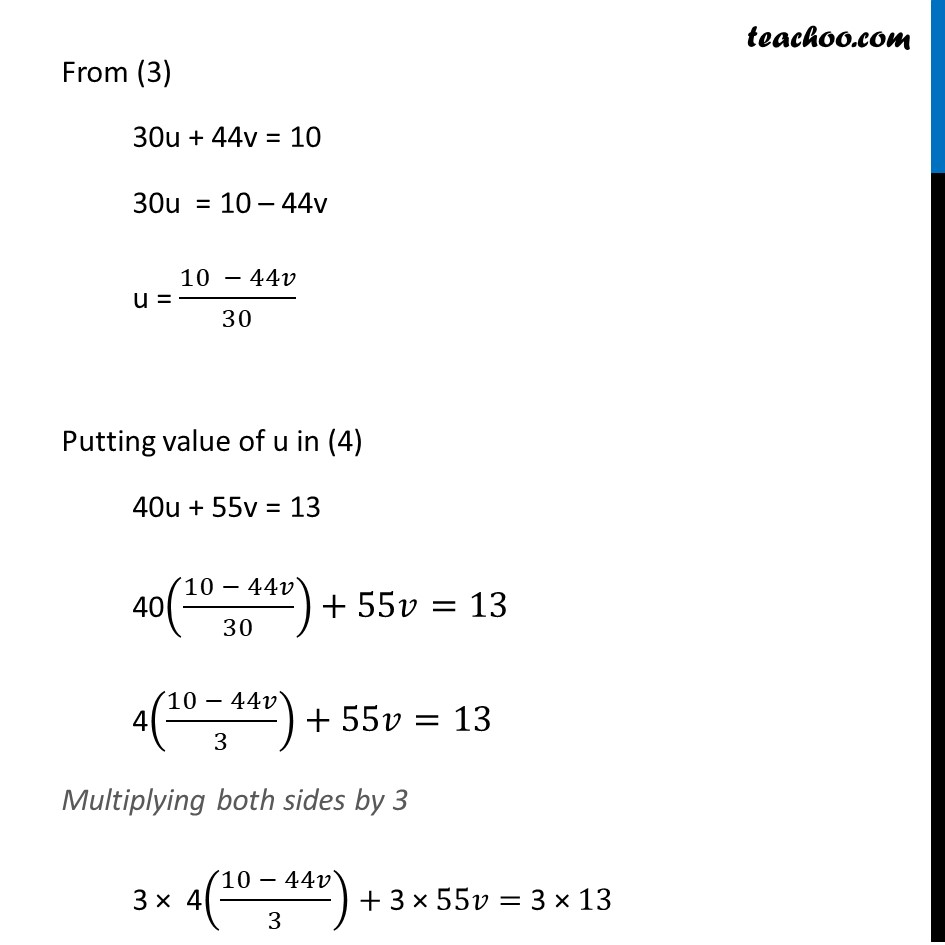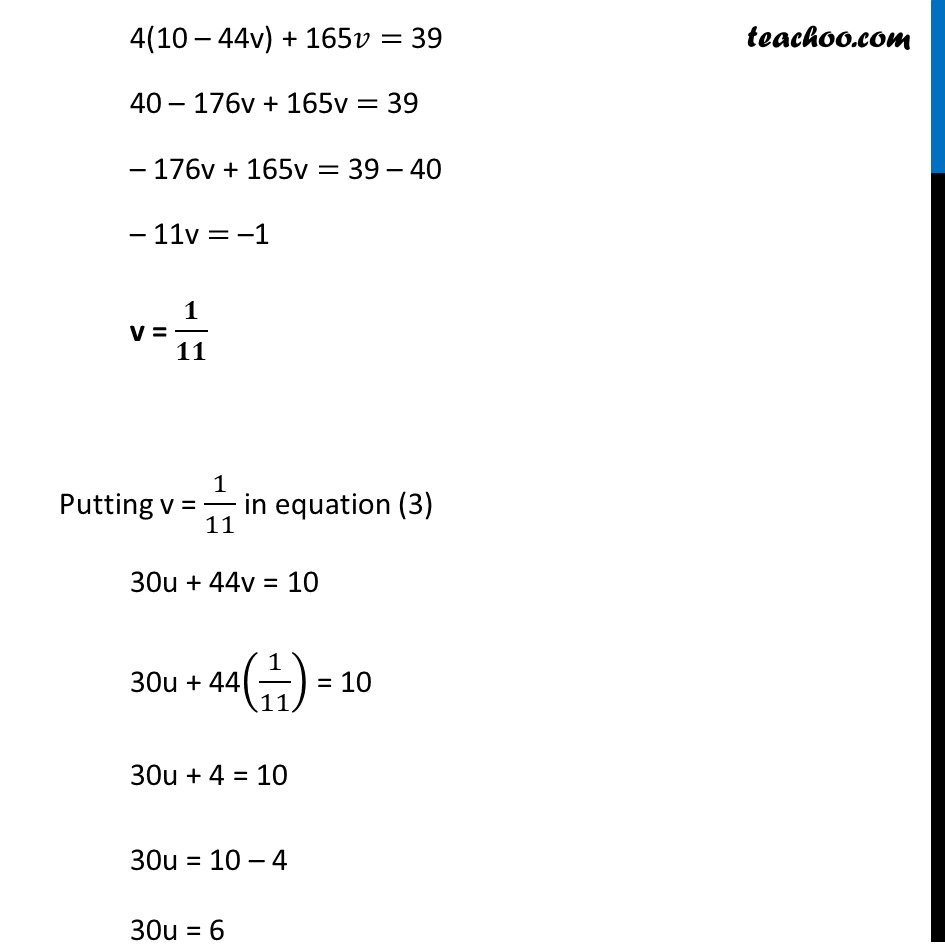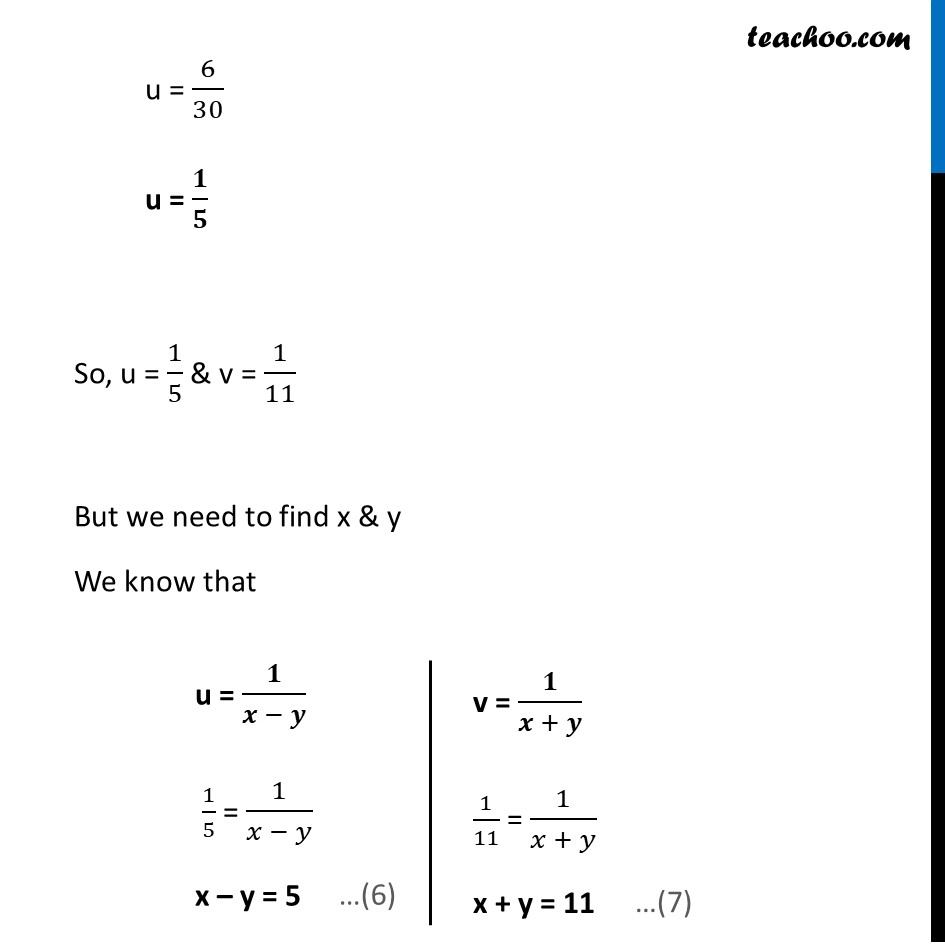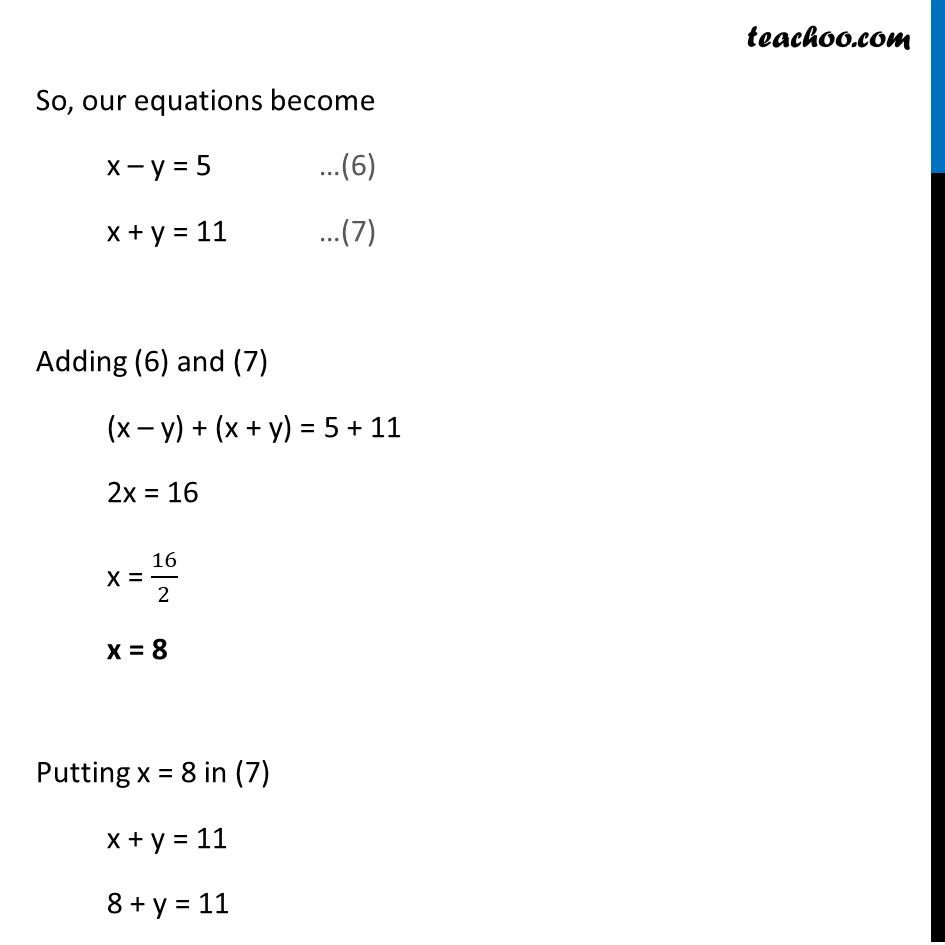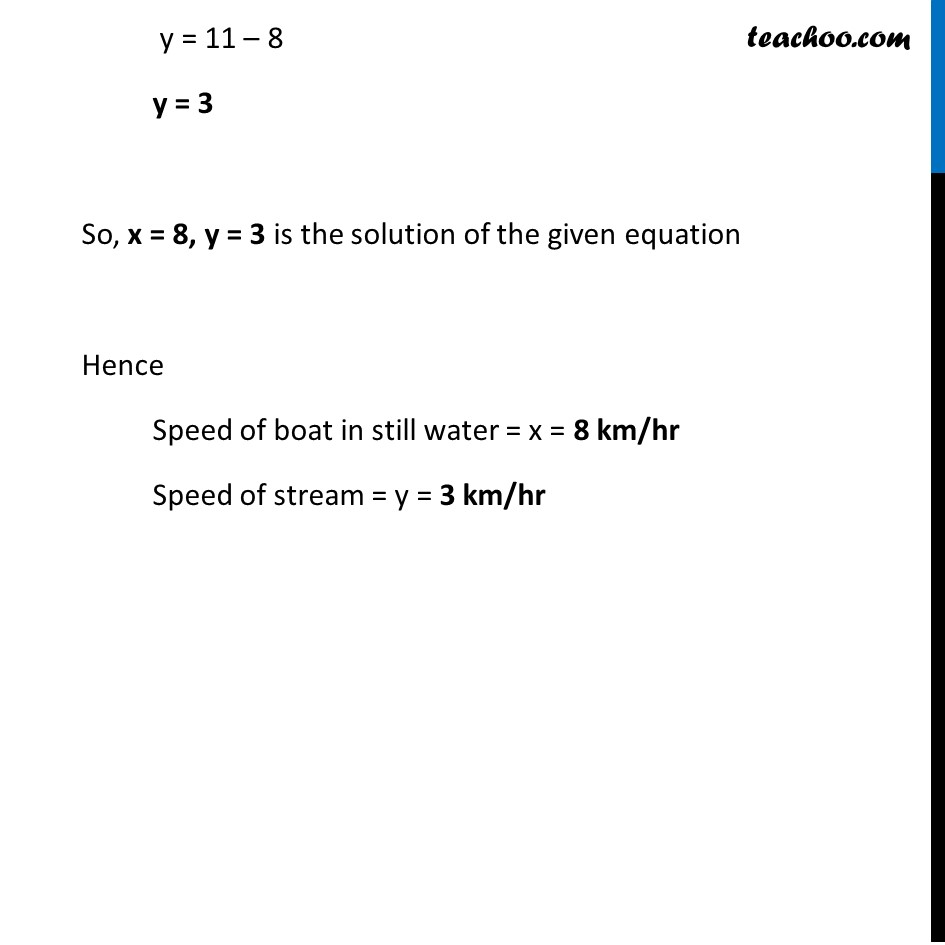Learn in your speed, with individual attention - Teachoo Maths 1-on-1 Class

### Transcript

Question 9 A boat goes 30 km upstream and 44 km downstream in 10 hours. In 13 hours, it can go 40 km upstream and 55 km down-stream. Determine the speed of the stream and that of the boat in still water. Let the speed of boat in still water be x km/hr & let the speed of stream be y km/hr Now, Speed downstream = x + y Speed upstream = x – y Given that A boat goes 30 km upstream and 44 km downstream in 10 hours Time taken to go 30 km upstream + Time taken to go 44 km downstream (𝐷𝑖𝑠𝑡𝑎𝑛𝑐𝑒 𝑜𝑓 30 𝑘𝑚)/(𝑆𝑝𝑒𝑒𝑑 𝑢𝑝𝑠𝑡𝑟𝑒𝑎𝑚) + (𝐷𝑖𝑠𝑡𝑎𝑛𝑐𝑒 𝑜𝑓 44 𝑘𝑚)/(𝑆𝑝𝑒𝑒𝑑 𝑑𝑜𝑤𝑛𝑠𝑡𝑟𝑒𝑎𝑚) = 10 𝟑𝟎/(𝒙 − 𝒚) + 𝟒𝟒/(𝒙 + 𝒚) = 10 Similarly, A boat goes 40 km upstream and 55 km downstream in 13 hours Time taken to go 40 km upstream + Time taken to go 55 km downstream (𝐷𝑖𝑠𝑡𝑎𝑛𝑐𝑒 𝑜𝑓 40 𝑘𝑚)/(𝑆𝑝𝑒𝑒𝑑 𝑢𝑝𝑠𝑡𝑟𝑒𝑎𝑚) + (𝐷𝑖𝑠𝑡𝑎𝑛𝑐𝑒 𝑜𝑓 55 𝑘𝑚)/(𝑆𝑝𝑒𝑒𝑑 𝑑𝑜𝑤𝑛𝑠𝑡𝑟𝑒𝑎𝑚) = 13 𝟒𝟎/(𝒙 − 𝒚) + 𝟓𝟓/(𝒙 + 𝒚) = 13 Our equations are 30 (1/(𝑥 − 𝑦))+44(1/(𝑥 + 𝑦))=10 …(1) 40 (1/(𝑥 − 𝑦))+55(1/(𝑥 + 𝑦))=13 …(2) So, our equations become 30u + 44v = 10 40u + 55v = 13 Solving 30u + 44v = 10 …(3) 40u + 55v = 13 …(4) From (3) 30u + 44v = 10 30u = 10 – 44v u = (10 − 44𝑣)/30 Putting value of u in (4) 40u + 55v = 13 40((10 − 44𝑣)/30)+55𝑣=13 4((10 − 44𝑣)/3)+55𝑣=13 Multiplying both sides by 3 3 × 4((10 − 44𝑣)/3)+"3 ×" 55𝑣="3 ×" 13 4(10 – 44v) + 165𝑣= 39 40 – 176v + 165v = 39 – 176v + 165v = 39 – 40 – 11v = –1 v = 𝟏/𝟏𝟏 Putting v = 1/11 in equation (3) 30u + 44v = 10 30u + 44(1/11) = 10 30u + 4 = 10 30u = 10 – 4 30u = 6 u = 6/30 u = 𝟏/𝟓 So, u = 1/5 & v = 1/11 But we need to find x & y We know that u = 𝟏/(𝒙 − 𝒚) 1/5 = 1/(𝑥 − 𝑦) x – y = 5 v = 𝟏/(𝒙 + 𝒚) 1/11 = 1/(𝑥 + 𝑦) x + y = 11 So, our equations become x – y = 5 …(6) x + y = 11 …(7) Adding (6) and (7) (x – y) + (x + y) = 5 + 11 2x = 16 x = 16/2 x = 8 Putting x = 8 in (7) x + y = 11 8 + y = 11 y = 11 – 8 y = 3 So, x = 8, y = 3 is the solution of the given equation Hence Speed of boat in still water = x = 8 km/hr Speed of stream = y = 3 km/hr